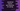# 3 different ways to remove the last character of a string in JavaScript## Introduction :

In this post, I will show you three different JavaScript programs to remove the last character of a string. For example, if the string is hello1, it will print hello. Try to run these examples and drop one comment below if you have any queries.

### 1. Using substring() :

The substring() function can extract one sub-string from a string by using the start index and length. We can pass the start index and the length of the sub-string as its first and the second parameter.

The syntax of substring() function is as below :

``str.substring(from: number, length?: number)``

Our problem is to remove the last character of a string. So, we can pass 0 as the first index and string-length - 1 as the length. For string hello1, if the first index is 0 and length is 5. It will return the sub-string from index 0 with length 5 i.e. hello.

length property of a string returns its length. Below is the complete program :

``````let givenStr = "Hello1";

console.log(givenStr.substr(0, givenStr.length - 1));``````

It will print :

``Hello``

### 2. Using slice :

The slice function extracts a section of a string. It extracts and returns a new string. It’s syntax is as below :

``str.slice(startIndex[, endIndex])``

To remove the last character, we can pass 0 as the first index and length - 1 as the end index.

``````let givenStr = "Hello1";

console.log(givenStr.slice(0, givenStr.length - 1));``````

Or, we can pass -1 as the end index. End index -x is treated as string-length - x.

``````let givenStr = "Hello1";

console.log(givenStr.slice(0, -1));``````

Both examples will print Hello.

### 3. Using replace :

replace() function takes two arguments. The first one is a regular expression that will find the sub-string to replace and the second one is the new substring to replace with.

``````let givenStr = "Hello1";

console.log(givenStr.replace(/.\$/,''));``````

Here, \$ is the regex pattern. It selects the last character of a string. The second parameter is empty. We are replacing the last character with that empty character. It will print Hello on the console.